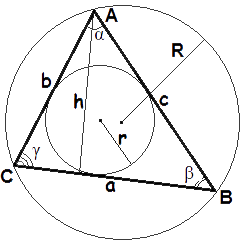## Ultimate Triangle Calculator

 Length of Side a: Length of Side b: Length of Side c: Angle A: degree radian mil sign second minute gon Angle B: degree radian mil sign second minute gon Angle C: degree radian mil sign second minute gon
Results:
 Area: Perimeter: Circumscribed Circle Radius: Inscribed Circle Radius: Height of a: Height of b: Height of c: Angle Bisector of a: Angle Bisector of b: Angle Bisector of c: Median of a: Median of b: Median of c:

Input The coordinates of the three points of the triangle calculate:

 X Y A : B : C :

The utlimate triangle calculator can solve all of triangle problems. The triangle is a basic shapes in geometry, We always write it as △ABC. It has three vertices A, B, and C and three sides.The Ultimate triangle calculator is very powerful. When you have 3 parameters of triangle 1. all 3 sides, 2. side-angle-side, 3. angle-side-angle, 4.all 3 angles, type them in, then you will get area, perimeter, Circumscribed Circle Radius, Inscribed Circle Radius, and so on.

For example, Length of Sides a = 3, b = 4, c = 5, then The calculator will give the result:

Angles A = 36.8699, B = 53.1301, C = 90. Area = 6, Perimeter = 12